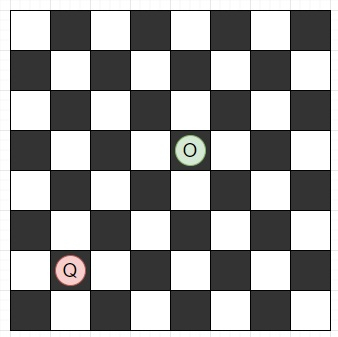# Check if a Queen can attack a given cell on chessboard in Python

PythonServer Side ProgrammingProgramming

Suppose we have two coordinates on a chessboard for queen and opponent. These points are Q and O respectively. We have to check whether the queen can attack the opponent or not. As we know that the queen can attack in the same row, same column and diagonally.

So, if the input is like Q = (1, 1) O = (4, 4), then the output will be True as Q can go (4, 4) diagonally.To solve this, we will follow these steps −

• if x of Q is same as x of O, then
• return True
• if y of Q is same as y of O, then
• return True
• if |x of Q - x of O| is same as |y of Q - y of O|, then
• return True
• return False

Let us see the following implementation to get better understanding −

## Example

Live Demo

def solve(Q, O):
if Q == O:
return True
if Q == O:
return True
if abs(Q - O) == abs(Q - O):
return True
return False
Q = (1, 1)
O = (4, 4)
print(solve(Q, O))

## Input

(1, 1), (4, 4)

## Output

True